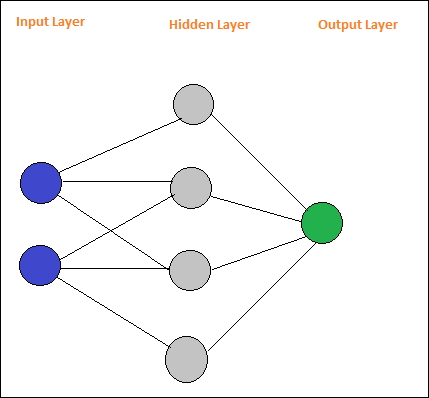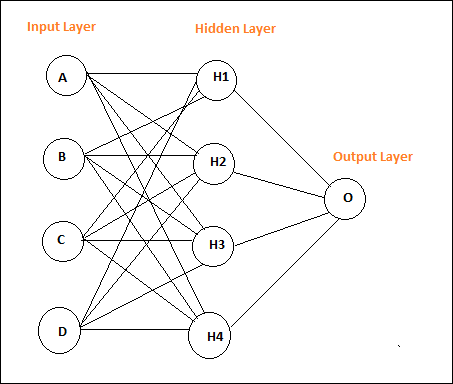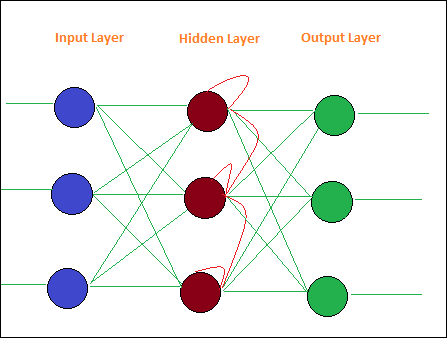# PyBrain - Introduction to PyBrain Networks

PyBrain is a library developed for Machine Learning with Python. There are some important concepts in Machine Learning and one among them is Networks. A network is composed of modules and they are connected using connections.

A layout of a simple neural network is as follows −Pybrain supports neural networks such as Feed-Forward Network, Recurrent Network, etc.

A feed-forward network is a neural network, where the information between nodes moves in the forward direction and will never travel backward. Feed Forward network is the first and the simplest one among the networks available in the artificial neural network. The information is passed from the input nodes, next to the hidden nodes and later to the output node.

Here is a simple feed forward network layout.The circles are said to be modules and the lines with arrows are connections to the modules.

The nodes A, B, C and D are input nodes

H1, H2, H3, H4 are hidden nodes and O is the output.

In the above network, we have 4 input nodes, 4 hidden layers and 1 output. The number of lines shown in the diagram indicate the weight parameters in the model that are adjusted during training.

Recurrent Networks are similar to Feed Forward Network with the only difference that it has to remember the data at each step. The history of each step has to be saved.

Here is a simple Layout of Recurrent Network −